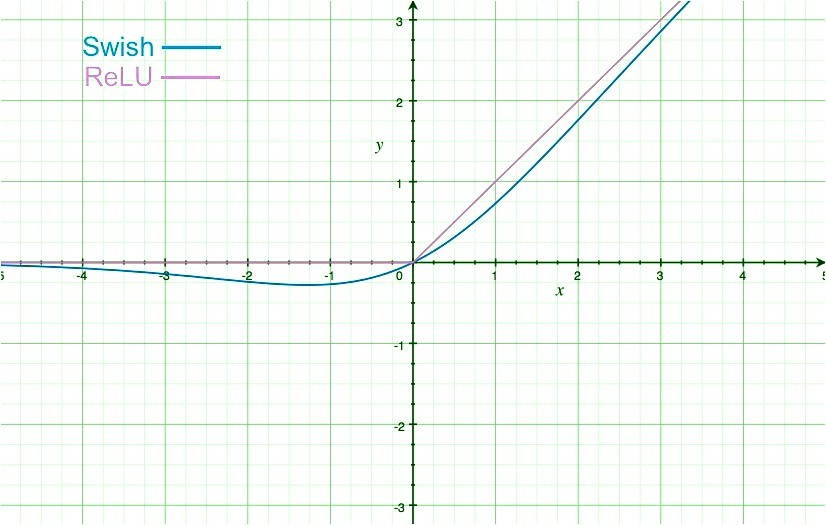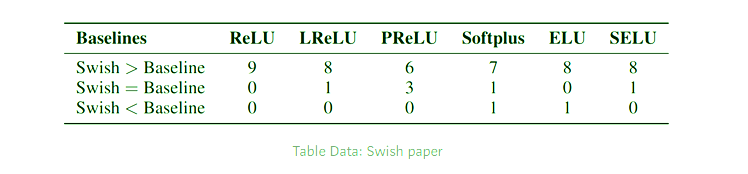# ML – Swish Function by Google in Keras

ReLU has been the best activation function in the deep learning community for a long time, but Google’s brain team announced Swish as an alternative to ReLU in 2017. Research by the authors of the papers shows that simply be substituting ReLU units with Swish units improves the classification accuracy on ImageNet by 0.6% for Inception-ResNet-v2, hence, it outperforms ReLU in many deep neural nets.

Swish Activation function:

• Mathematical formula: Y = X * sigmoid(X)
• Bounded below but Unbounded above: Y approach to constant value at X approaches negative infinity but Y approach to infinity as X approaches infinity.
• Derivative of Swish, Y’ = Y + sigmoid(X) * (1-Y)
• Soft curve and non-monotonic function.Swish vs ReLU

Having no bounds is desirable for activation functions as it avoids problems when gradients are nearly zero. The ReLU function is bounded above but when we consider the below region then being bounded below may regularize the model up to an extent, also functions that approach zero in a limit to negative infinity are great at regularization because large negative inputs are discarded. The swish function provides it along with being non-monotonous which enhances the expression of input data and weight to be learnt.
Below is the performance metric of Swish function over many community dominant activation functions like ReLU, SeLU, Leaky ReLU and others.Implementation of Swish activation function in keras:
Swish is implemented as a custom function in Keras, which after defining has to be registered with a key in the Activation Class.

Code:

 `# Code from between to demonstrate the implementation of Swish ` ` `  `# Our aim is to use "swish" in place of "relu" and make compiler understand it ` `model.add(Dense(``64``, activation ``=` `"relu"``)) ` `model.add(Dense(``16``, activation ``=` `"relu"``)) `

Now We will be creating a custom function named Swish which can give the output according to the mathematical formula of Swish activation function as follows:

 `# Importing the sigmoid function from ` `# Keras backend and using it ` `from` `keras.backend ``import` `sigmoid ` ` `  `def` `swish(x, beta ``=` `1``): ` `    ``return` `(x ``*` `sigmoid(beta ``*` `x)) `

Now as we have the custom-designed function which can process the input as Swish activation, we need to register this custom object with Keras. For this, we pass it in a dictionary with a key of what we want to call it and the activation function for it. The Activation class will actually build the function.

Code:

 `# Getting the Custom object and updating them ` `from` `keras.utils.generic_utils ``import` `get_custom_objects ` `from` `keras.layers ``import` `Activation ` ` `  `# Below in place of swish you can take any custom key for the name  ` `get_custom_objects().update({``'swish'``: Activation(swish)}) `

Code: Implementing the custom-designed activation function

 `model.add(Dense(``64``, activation ``=` `"swish"``)) ` `model.add(Dense(``16``, activation ``=` `"swish"``)) `

My Personal Notes arrow_drop_upCheck out this Author's contributed articles.

If you like GeeksforGeeks and would like to contribute, you can also write an article using contribute.geeksforgeeks.org or mail your article to contribute@geeksforgeeks.org. See your article appearing on the GeeksforGeeks main page and help other Geeks.

Please Improve this article if you find anything incorrect by clicking on the "Improve Article" button below.

Article Tags :
Practice Tags :

Be the First to upvote.

Please write to us at contribute@geeksforgeeks.org to report any issue with the above content.